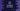# Java Program to find Transpose of a matrix### Java program to Transpose a matrix :

In this tutorial, we will learn how to print the transpose of a matrix in Java. But first let me show you want is transpose of a Matrix :

### What is Transpose of a Matrix :

Transpose of a matrix is a matrix whose rows are columns of the original one . That means, columns are rows of the original matrix.

For example :

``````1 2 3
4 5 6
7 8 9``````

The transpose of this above matrix is :

``````1 4 7
2 5 8
3 6 9``````

### Java program to print the transpose of a Matrix :

1. First we will take the inputs of the matrix using two ‘for’ loops
2. We will scan the matrix using two ‘for’ loops and print it out as column wise i.e. first column as first row, second column as second row etc.
``````import java.util.Scanner;

public class Main {

/**
* Utility functions for System.out.println() and System.out.print()
*/
private static void print(String str) {
System.out.print(str);
}

private static void println(String str) {
System.out.println(str);
}

/**
* Print one matrix in normal order
*
* @param matrix : Matrix to print
* @param row    : total row count
* @param column : total column count
*/
private static void printMatrix(int[][] matrix, int row, int column) {
for (int i = 0; i < row; i++) {
for (int j = 0; j < column; j++) {
print(matrix[i][j] + " ");
}
println("");
}
}

/**
* Print Transpose matrix
*
* @param matrix : Matrix to print
* @param row    : total row count
* @param column : total column count
*/
private static void printTransposeMatrix(int[][] matrix, int row, int column) {
for (int i = 0; i < column; i++) {
for (int j = 0; j < row; j++) {
print(matrix[j][i] + " ");
}
println("");
}
}

public static void main(String args[]) {
int i, j;

Scanner scanner = new Scanner(System.in);

println("Enter total number of rows of the matrix : ");
int row = scanner.nextInt();

println("Enter total number of columns of the matrix : ");
int column = scanner.nextInt();

int matrix[][] = new int[row][column];

println("Enter the matrix elements one by one : ");

//get the matrix input from the user
for (i = 0; i < row; i++) {
for (j = 0; j < column; j++) {
matrix[i][j] = scanner.nextInt();
}
}

//print the matrix
println("Matrix you have entered : ");
printMatrix(matrix, row, column);

//print the matrix as transpose
println("Transpose matrix of the above is : ");
printTransposeMatrix(matrix, row, column);
}
}``````

### Sample output :

``````Enter total number of rows of the matrix :
3
Enter total number of columns of the matrix :
3
Enter the matrix elements one by one :
1
4
6
7
9
10
11
14
15
Matrix you have entered :
1 4 6
7 9 10
11 14 15
Transpose matrix of the above is :
1 7 11
4 9 14
6 10 15 ``````